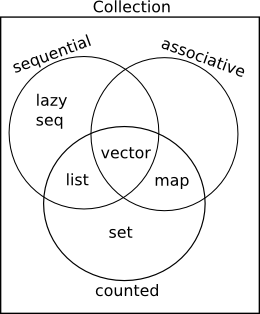## 数据结构

### Collection 和 Sequence

Clojure 常用的数据结构有 List, Map, Vector, Set.Clojure 中的数据结构被设计成不可变的, 用来配合函数式编程. 这和主流编程语言存在区别.

### Sequence & List

Clojure 中的 List 是单链表. 适合从头部访问, 而不适合随机访问. Clojure 采用 Lisp 语法来定义 List:

'(1 2 3 4); (1 2 3 4)(list? '(1 2 3 4)); true

(quote (1 2 3 4)); (1 2 3 4)

(list 1 2 3 4); (1 2 3 4)

Sequence 是一种抽象的数据结构, List 本身是一种 Sequence, 同时 Sequence 本身可以表示惰性的列表.

(type (range)); cljs.core/Range(seq? (range)); true

(seq [1 2 3]); (1 2 3)

(first (list 1 2 3 4)); 1(rest (list 1 2 3 4)); (2 3 4)(cons 1 '(2 3 4 5 6)); (1 2 3 4 5 6)

### Vector

Vector 支持随机访问, 通过 [] 语法可以快速定义:

[1 2 3 4]; [1 2 3 4](vector? []); true

(vector 1 2 3 4); [1 2 3 4](vec '(1 2 3 4)); [1 2 3 4]

(get [:a :b :c :d] 1); :b(nth [:a :b :c :d] 1); :b(peek [1 2 3 4]); 4(pop [1 2 3]); [1 2](conj [1 2 3] 4); [1 2 3 4](subvec [1 2 3] 1 2) ; 截取向量; 

### Map

Map 是关联列表, 可以通过 {} 语法定义 Map, 其中 , 等同于空白. 一般键和值的类型没有具体限制:

{:a "a", "b" 3, 4 []}; {:a "a", "b" 3, 4 []}(map? {}); true

{:name "Clojure", :born 2007}

(hash-map 1 2 3 4); {1 2, 3 4}(zipmap [:a :b :c] [1 2 3]); {:a 1, :b 2, :c 3}

(:a {:a 1}); 1(assoc {:a 1, :b 2} :c 3); {:a 1, :b 2, :c 3}(dissoc {:a 1} :b 2); {:a 1}(contains? {:a 1} :b); false(merge {:a 1} {:b 2}); {:a 1, :b 2}(update {:a 1} :a inc); {:a 2}

### Set

#{1 2 3}; #{1 2 3}(set? #{}); true

(hash-set 1 2 3); #{1 2 3}(set [1 2 3]); #{1 2 3}

(conj #{1 2 3} 4); #{1 2 3 4}(disj #{1 2 3} 3); #{1 2}(contains? #{1 2 3} 4); false

### 常用函数

• into

(into [] '(1 2 3)); [1 2 3](into #{} '(1 2 3)); #{1 2 3}(into '() [1 2 3]); (3 2 1)(into {} [[:a 1] [:b 2]]); {:a 1, :b 2}
• first

first 取出 Sequence 的第一个元素, 但是对于 Map 来说能获取键值对:

(first [1 2 3]); 1(first '(1 2 3)); 1(first #{1 2 3}); 1(first {:a 1 :b 2}); [:a 1]
• map

(map inc [1 2 3]); (2 3 4)(map inc '(1 2 3)); (2 3 4)(map inc #{1 2 3}); (2 3 4)(map identity {:a 1 :b 2}); ([:a 1] [:b 2])

(mapv identity {:a 1 :b 2}); [[:a 1] [:b 2]](into [] (map identity {:a 1 :b 2})); [[:a 1] [:b 2]]
• peek, popconj

(peek [1 2 3]); 3(peek '(1 2 3)); 1(pop '(1 2 3)); (2 3)(pop [1 2 3]); [1 2](conj [1 2 3] 4); [1 2 3 4](conj '(1 2 3) 4); (4 1 2 3)
• count
(count '(1 2 3)); 3(count [1 2 3]); 3(count "123"); 3(count #{1 2 3}); 3(count {:a 1 :b 2 :c 3}); 3

(empty? []); true(empty? {}); true(empty? #{}); true(empty? '()); true
• assoc-inupdate-in

(get-in {:a [1 2 3]} [:a 0]); 1(assoc-in {:a [1 2 3]} [:a 0] 4); {:a [4 2 3]}(update-in {:a [1 2 3]} [:a 0] inc); {:a [2 2 3]}